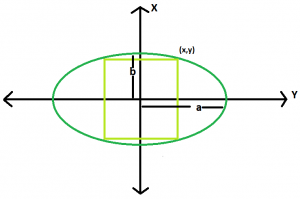GeeksforGeeks App
Open AppBrowser
Continue

# Area of the Largest square that can be inscribed in an ellipse

Given an ellipse, with major axis length 2a & 2b, the task is to find the area of the largest rectangle that can be inscribed in it.
Examples:

```Input: a = 4, b = 2
Output: 1.25

Input: a = 5, b= 3
Output: 0.604444```Approach: If a square is inscribed in an ellipse, the distance from the centre of the square to any of its corners will be equal to the distance between the origin and the point on the upper right corner in the diagram below, where x=y

the equation of the ellipse is x^2/a^2 + y^2/b^2 = 1
If, x = y
then, x^2/a^2 + x^2/b^2 = 1
therefore, x = √(a^2 + b^2)/ab
so, y = √(a^2 + b^2)/ab
So Area, A = 4(a^2 + b^2)/a^2b^2

Below is the implementation of above approach:

## C++

 `// C++ Program to find the biggest square``// which can be inscribed within the ellipse``#include ``using` `namespace` `std;` `// Function to find the area``// of the square``float` `squarearea(``float` `a, ``float` `b)``{` `    ``// a and b cannot be negative``    ``if` `(a < 0 || b < 0)``        ``return` `-1;` `    ``// area of the square``    ``float` `area = 4 * ((``pow``(a, 2) + ``pow``(b, 2))``                      ``/ (``pow``(a, 2) * ``pow``(b, 2)));` `    ``return` `area;``}` `// Driver code``int` `main()``{``    ``float` `a = 4, b = 2;``    ``cout << squarearea(a, b) << endl;` `    ``return` `0;``}`

## Java

 `// Java Program to find the biggest square``// which can be inscribed within the ellipse``import` `java.io.*;` `class` `GFG {`  `// Function to find the area``// of the square``static` `float` `squarearea(``float` `a, ``float` `b)``{` `    ``// a and b cannot be negative``    ``if` `(a < ``0` `|| b < ``0``)``        ``return` `-``1``;` `    ``// area of the square``    ``float` `area = ``4` `*(``float``) ((Math.pow(a, ``2``) + Math.pow(b, ``2``))``                    ``/ (Math.pow(a, ``2``) * Math.pow(b, ``2``)));` `    ``return` `area;``}` `// Driver code` `    ``public` `static` `void` `main (String[] args) {``        ``float` `a = ``4``, b = ``2``;``    ``System.out.println( squarearea(a, b));` `    ``}``}``// This code is contributed by inder_verma.`

## Python 3

 `# Python3 Program to find the biggest square``# which can be inscribed within the ellipse`  `# Function to find the area``# of the square``def` `squarearea( a, b):`  `    ``# a and b cannot be negative``    ``if` `(a < ``0` `or` `b < ``0``):``        ``return` `-``1``    `  `    ``# area of the square``    ``area ``=` `4` `*` `(((``pow``(a, ``2``) ``+` `pow``(b, ``2``)) ``/``               ``(``pow``(a, ``2``) ``*` `pow``(b, ``2``))))` `    ``return` `area`  `# Driver code``if` `__name__``=``=``'__main__'``:``    ``a ``=` `4``    ``b ``=` `2``    ``print``(squarearea(a, b))` `# This code is contributed by ash264`

## C#

 `// C# Program to find the biggest``// square which can be inscribed``// within the ellipse``using` `System;` `class` `GFG``{` `// Function to find the area``// of the square``static` `float` `squarearea(``float` `a, ``float` `b)``{` `    ``// a and b cannot be negative``    ``if` `(a < 0 || b < 0)``        ``return` `-1;` `    ``// area of the square``    ``float` `area = 4 *(``float``) ((Math.Pow(a, 2) +``                              ``Math.Pow(b, 2)) /``                             ``(Math.Pow(a, 2) *``                              ``Math.Pow(b, 2)));` `    ``return` `area;``}` `// Driver code``public` `static` `void` `Main ()``{``    ``float` `a = 4, b = 2;``    ``Console.WriteLine( squarearea(a, b));``}``}` `// This code is contributed by inder_verma`

## PHP

 ``

## Javascript

 ``

Output:

`1.25`

Time complexity: O(1)

Auxiliary Space: O(1)

My Personal Notes arrow_drop_up